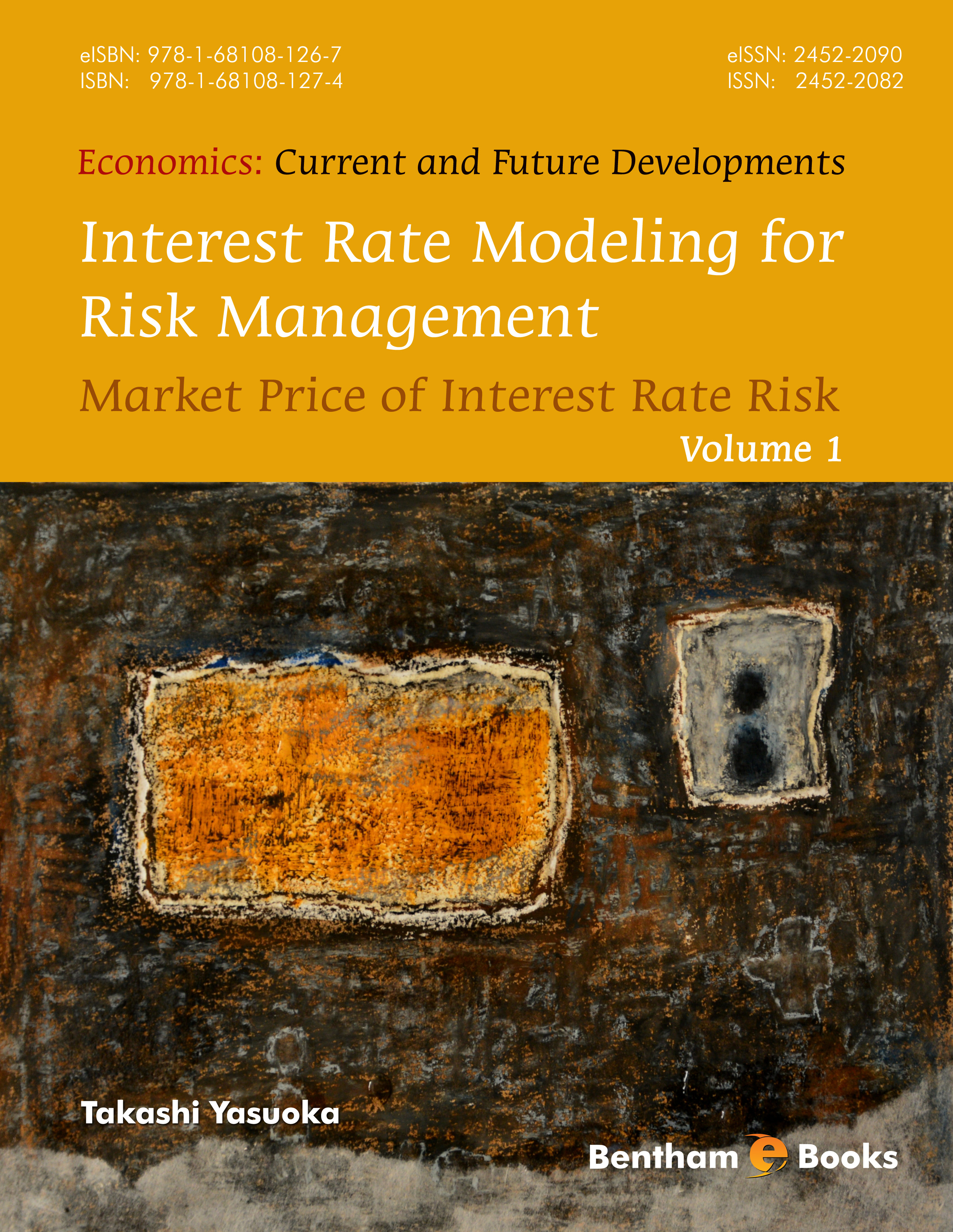Author: Takashi Yasuoka

### Volume 1

eBook: US \$89 Special Offer (PDF + Printed Copy): US \$163
Printed Copy: US \$119
ISSN: 2452-2082 (Print)
ISSN: 2452-2090 (Online)
ISBN: 978-1-68108-127-4 (Print)
ISBN: 978-1-68108-126-7 (Online)
Year of Publication: 2015
DOI: 10.2174/97816810812671150101

#### Introduction

Interest Rate Modeling for Risk Management addresses interest rate modeling for risk management. The interest rate model is specified under the real-world measure, and the result is used as to generate scenarios for interest rates. This type of system is referred to as ‘real-world model’ in this book. The book introduces a theoretical framework that allows estimating the market price of interest rate risk. For this, the book starts with a brief explanation of stochastic analysis, and introduces interest rate models such as Heath-Jarrow-Morton, Hull-White and LIBOR models. The real-world model is then introduced in subsequent chapters. Additionally, the book also explains some properties of the real-world model, along with the negative price tendency of the market price for risk and a positive market price of risk (with an example of this actually occurring). Readers will also find a handy appendix with proofs to complement the numerical methods explained in the book.

This book is intended as a primer for practitioners in financial institutions involved in interest rate risk management. It also presents a new perspective for researchers and graduates in econometrics and finance on the study of interest rate models.

Indexed in: Book Citation Index, Social Sciences & Humanities, EBSCO.

# Preface

Interest rate risk is one of the most important types of financial risk that must be considered by banks, insurers, and other financial institutions. Typically, an empirical scenario model of interest rates has been used to measure risk. However, interest rate models are a promising tool for generating the scenarios. To develop this topic, this book introduces advanced methods for employing interest rate models as scenario models.

An interest rate model describes the dynamical behavior of interest rates by a stochastic differential equation. Initially, this behavior is specified under the real-world measure, with the result called a real-world model. The market price of risk is mathematically de ned so as to ensure the arbitrage-free property of the model. In practice, the model is represented under a risk-neutral measure to eliminate the market price of risk; we call the result a risk-neutral model. Interest rate models has been developed as risk-neutral models because of their convenience: the market price of risk does not need to be estimated for such models. Because of this, estimation of the market price of risk has not been theoretically studied for several decades.

On the one hand, if we are going to use interest rate models for risk management, then we should use real-world models. This means that we need to numerically obtain the market price of risk to work with real-world models. On the other hand, estimating the market price of risk has been, in practice, fraught with ambiguity. To address this, this book introduces a theory of real-world modeling based on recent results in my other works.

This book is intended primarily for practitioners in financial institutions. Additionally, this book presents a new perspective on the study of interestrate models because real-world modeling is an emerging subject for the future.This book contains a number of substantial and advanced subjects likely to be of interest to researchers and graduate students in financial engineering or econometrics.

This book consists of two main parts. The first part summarizes the fundamentals of interest rate models. Chapter 1 introduces the basic concepts of fixed income risk and interest rate risk. Chapter 2 introduces stochastic calculus and stochastic differential equations. Chapter 3 presents arbitrage pric ing theory, adopting the martingale approach, and denes the market price of risk. Chapter 4 introduces interest rate modeling in the Heath{Jarrow{Morton (HJM) framework. As the last topic in the rst part, the LIBOR market model is introduced in Chapter 5.

The second part introduces the theory of real-world models, methods of estimating the market price of risk, properties of the market price of risk, and numerical features of real-world simulation. I expect that the contents of this part will be helpful to those who need to use the real-world model in practice. Results in this second part are based mostly on my other works, to which this book serves as a complement, offering additional precision and more thorough explanations. Additionally, this book contains newly written content on topics including maximum likelihood estimation of the market price of risk, real-world modeling in the Hull{White model, and the negative price tendency of the market price of risk.

Chapter 6 develops the fundamental theory of the real-world model in the HJM model, and in Chapter 7 we give some remarks about the real-world model. Chapter 8 presents real-world modeling, focusing on the Hull{White model as an application of the results in the HJM model. Chapter 9 introduces the theory for the real-world model in the LIBOR market model, presenting the topics in parallel with the presentation in Chapter 6. The remarks given in Chapter 7 are also applicable to the real-world models in the Hull{White model and the LIBOR market model. As a conclusion, Chapter 10 shows some numerical examples that demonstrate results from Chapters 6 to 9. The Appendix presents some basic algorithms used in numerical analysis and the proofs of some of the results from Chapter 9.

Throughout this book, I have tried to describe the development of mathematical expression as straightforwardly as possible and have included figures to illustrate some points. Because of this, in some places strict mathematical style has been left aside to obtain practical implications.

So far, the real-world model has been only a dream with respect to practical use. My hope is that this book will help bring the model from dreams to the real world and provide worthwhile suggestions for reconsidering the current state of interest-rate-risk management.

Takashi Yasuoka